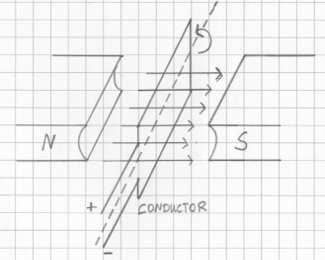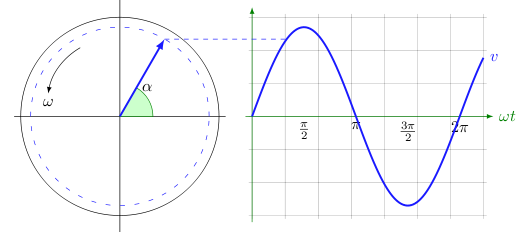# AC Voltage

Consider the badly hand-drawn image below:When the lines of a magnetic field are cut by a conductor, a voltage is induced. The strength of this voltage depends upon the rotational speed of the conductor, the number of turns in the conductor, and the strength of the magnetic field. Together the magnetic field and conductor give us a generator.

Keeping with the previous image, we'll say that the conductor is parallel to the lines of the magnetic field at 0 radians, and we see that it rotates to the left. The lines are cut more slowly at 0 radians than they are at π/2 radians (perpendicular to the lines). This means that the the voltage induced at 0 radians is less than the voltage induced at π/2 radians. In fact, at 0 radians the minimum voltage is induced, and at π/2 radians the maximum voltage is induced. At π radians the conductor is parallel to the lines again and the minimum voltage is induced. From π to 2π radians negative voltage is induced. This is all shown in the following graph:If v is the instantaneous voltage and V0 the peak voltage, then

```	v=V0sin(ωt)
```

## References

Fischer-Cripps. A.C., The Electronics Companion. Institute of Physics, 2005.